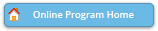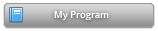#### Abstract Details

 Activity Number: 255 - Contributed Poster Presentations: Section on Statistical Computing Type: Contributed Date/Time: Monday, July 29, 2019 : 2:00 PM to 3:50 PM Sponsor: Section on Statistical Computing Abstract #301672 Title: Computational Effort of Multiple Hypothesis Testing Author(s): Georg Hahn* Companies: Keywords: multiple testing; hypothesis; sample; optimal allocation; runtime; computational effort Abstract: This poster is concerned with the optimal computational effort (i.e., allocation of Monte Carlo simulations) in multiple testing. In practice, p-values of all hypotheses are usually unknown and thus have to be approximated with Monte Carlo simulations. Consider the allocation of a pre-specified total integer number of simulations 'K' to a given finite number 'm' of hypotheses in order to approximate their p-values in an optimal way, in the sense that the allocation minimises the expected number of misclassified hypotheses (with respect to the correct decisions obtained if p-values were known). Under the assumption that the p-values are known and K is real-valued, and using a normal approximation of the Binomial distribution, the optimal real-valued allocation of K simulations to m hypotheses is derived when correcting for multiplicity with the Bonferroni correction, both when computing p-value estimates with or without a pseudo-count. Empirical evidence is given that the optimal integer and real-valued allocations are likely of the same form, that both seem to coincide asymptotically, and that a published multiple testing algorithm allocates simulations in a close-to-optimal way.

Authors who are presenting talks have a * after their name.